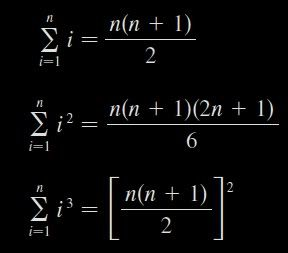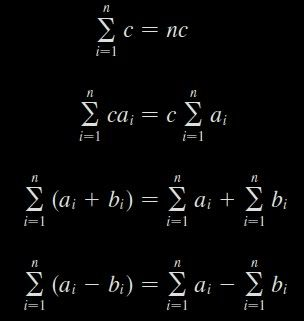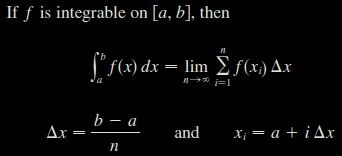# Stewart – Calculus – 5.1 / 5.2 – Areas and Distances / The Definite Integral

Approximating Area Using Rectangles

The Definite Integral

Calculating a Definite Integral Using Riemann Sums — Part 1

Calculating a Definite Integral Using Riemann Sums — Part 2

Three equations that give formulas for sums of powers of positive integers:These formulas are simple rules for working with sigma notation:Use the form of the definition of the integralto evaluate the integral.$\int_{1}^{2} x^3 dx$This site uses Akismet to reduce spam. Learn how your comment data is processed.